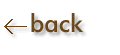Mathematics of Chemistry:

Mathematics is used widely in chemistry as well as all other sciences. Mathematical calculations are absolutely necessary to explore important concepts in chemistry. Without some basic mathematics skills, these calculations, and therefore chemistry itself, will be extremely difficult. However, with a basic knowledge of some of the mathematics that will be used in your chemistry course, you will be well prepared to deal with the concepts and theories of chemistry.
1. Mathematics Review
2. Calculator Fundamentals